Algebra 1 4-7 Complete Lesson: Arithmetic Sequences
starstarstarstarstarstarstarstarstarstar
by Matthew Richardson
| 42 Questions
Note from the author:
A complete formative lesson with embedded slideshow, mini lecture screencasts, checks for understanding, practice items, mixed review, and reflection. I create these assignments to supplement each lesson of Pearson's Common Core Edition Algebra 1, Algebra 2, and Geometry courses. See also mathquest.net and twitter.com/mathquestEDU.The outlined content above was added from outside of Formative.1
1
10 pts
Solve It! A wooden post-and-rail fence with two rails is made as shown. Find the number of pieces of wood needed to build a 4-section fence, a 5-section fence, and a 6-section fence. Not all items will be used.
• 13 pieces
• 16 pieces
• 19 pieces
• 20 pieces
• 24 pieces
• 25 pieces
• 26 pieces
• 27 pieces
• 4-section fence
• 5-section fence
• 6-section fence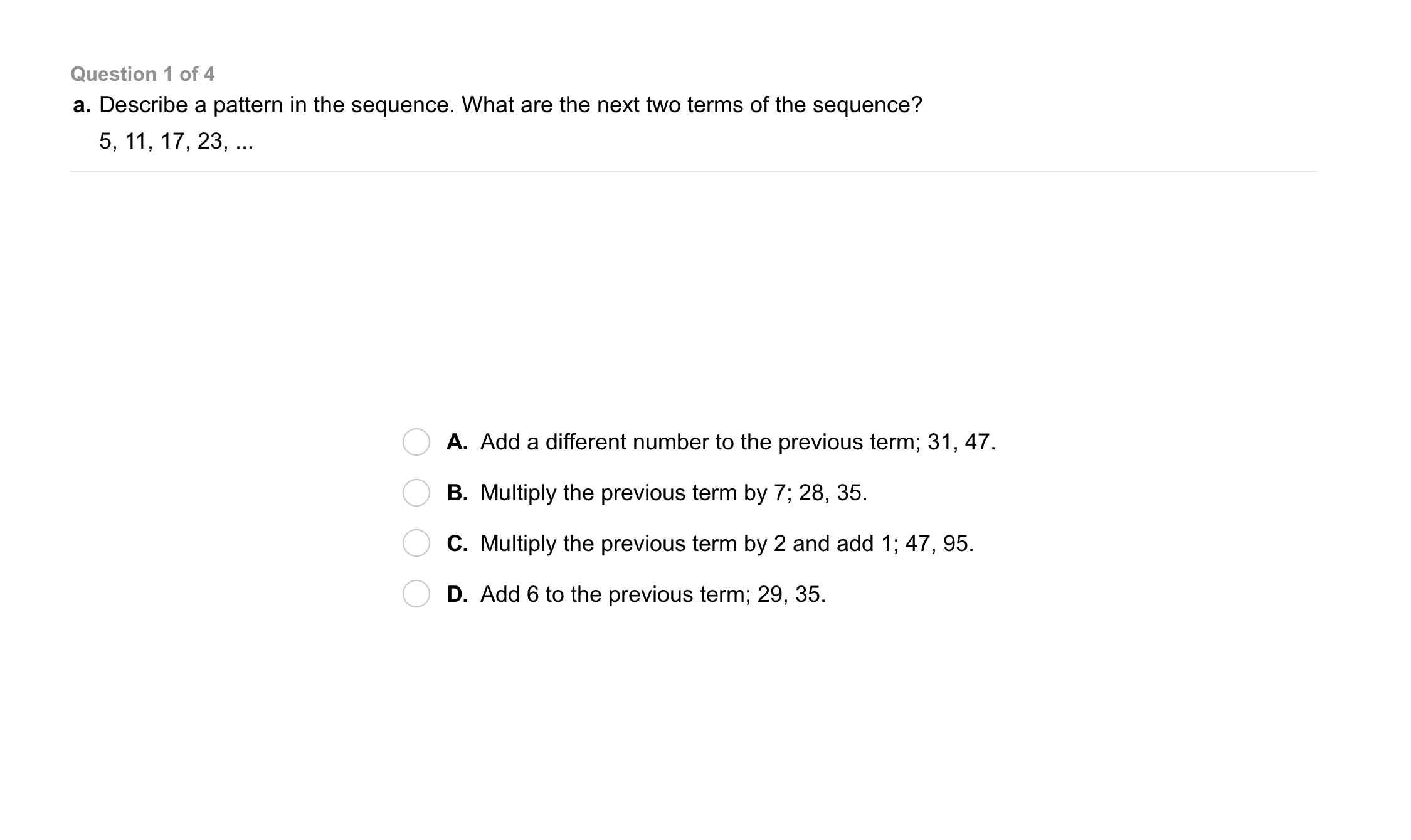2
2
10 pts
Problem 1 Got It?
A
B
C
D3
3
10 pts
Problem 1 Got It?
A
B
C
D4
4
10 pts
Problem 1 Got It?
A
B
C
D5
5
10 pts
Problem 1 Got It?
A
B
C
D6
6
10 pts
Problem 2 Got It?
A
B
C
D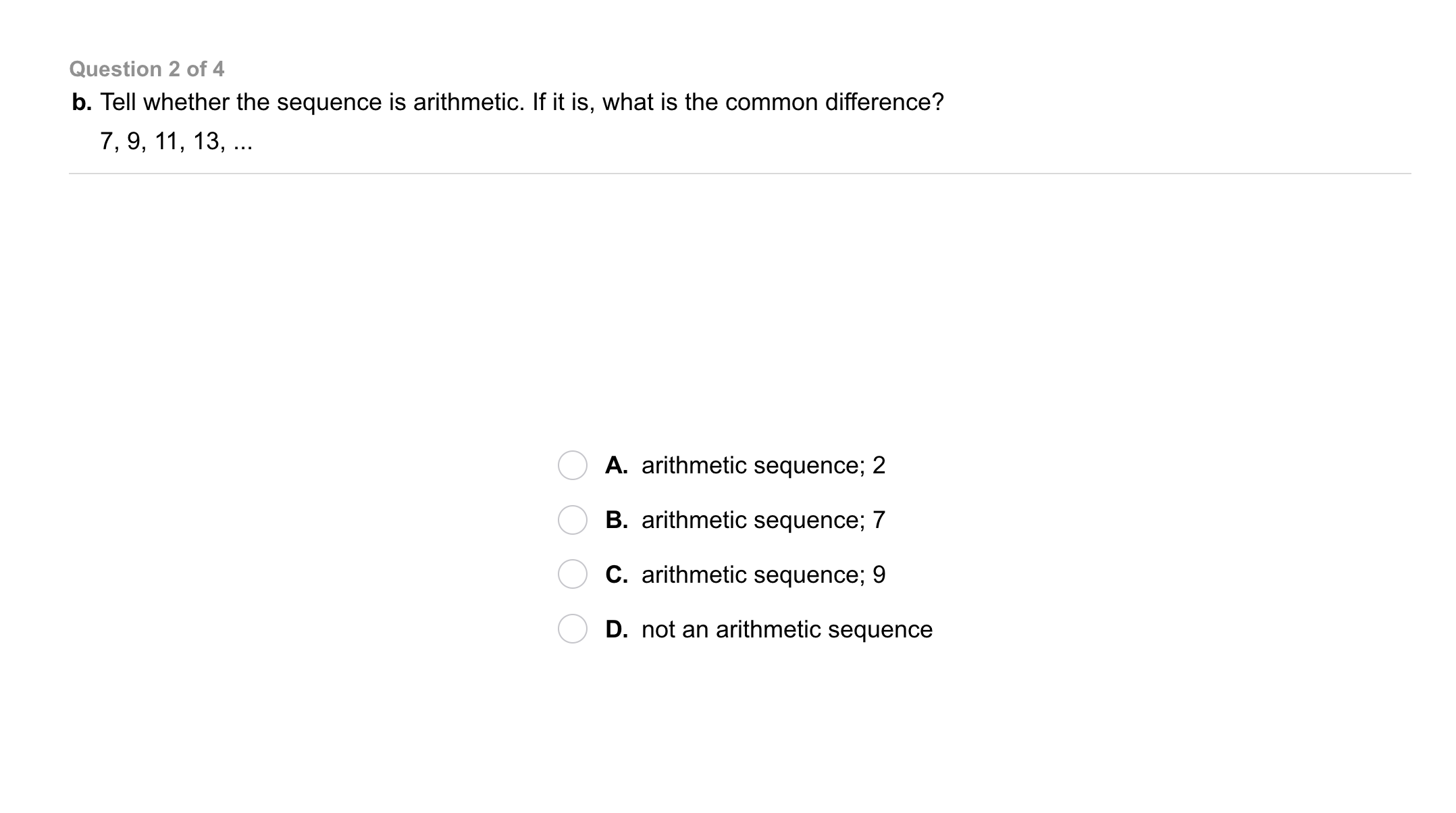7
7
10 pts
Problem 2 Got It?
A
B
C
D8
8
10 pts
Problem 2 Got It?
A
B
C
D9
9
10 pts
Problem 2 Got It?
A
B
C
D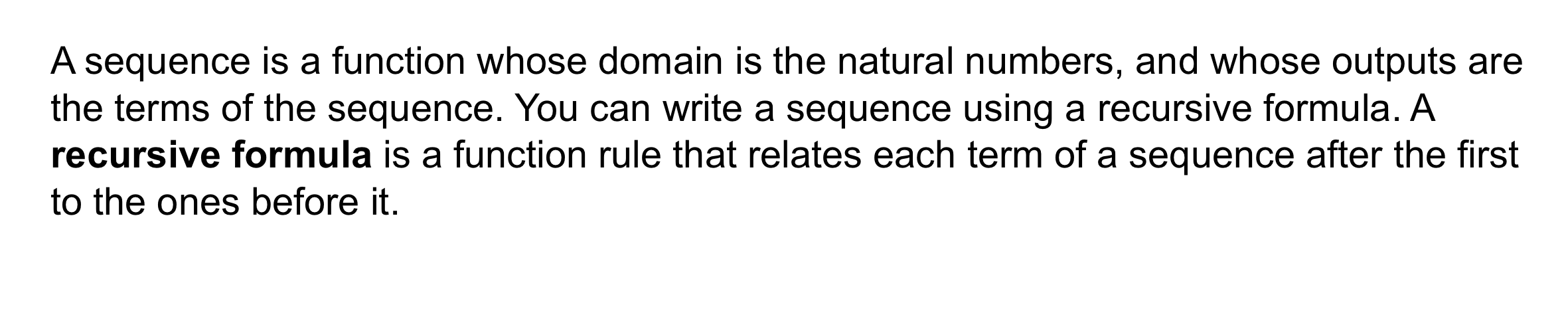10
10 pts
Problem 3 Got It? Write a recursive formula for the arithmetic sequence.

A(n) = A(n - 1) + 4; A(1) = 3
A(n) = A(n - 1) + 6; A(1) = 3
A(n) = A(n - 1) + 5; A(1) = 3
A(n) = A(n - 1) + 3; A(1) = 3
11
10 pts
Problem 3 Got It? What is the 9th term of the arithmetic sequence?

A(9) = 51
A(9) = 35
A(9) = 27
A(9) = 9
12
10 pts
Problem 3 Got It? Write a recursive formula for the arithmetic sequence.

A(n) = A(n - 1) + 15; A(1) = 23
A(n) = A(n - 1) + 12; A(1) = 23
A(n) = A(n - 1) + 18; A(1) = 23
A(n) = A(n - 1) + 3; A(1) = 23
13
10 pts
Problem 3 Got It? What is the 9th term of the arithmetic sequence?

A(9) = 81
A(9) = 151
A(9) = 107
A(9) = 119
14
10 pts
Problem 3 Got It? Write a recursive formula for the arithmetic sequence.

A(n) = A(n - 1) + 2.5; A(1) = 7.3
A(n) = A(n - 1) + 1.5; A(1) = 7.3
A(n) = A(n - 1) + 0.5; A(1) = 7.3
A(n) = A(n - 1) + 1; A(1) = 7.3
15
10 pts
Problem 3 Got It? What is the 9th term of the arithmetic sequence?

A(9) = 11.3
A(9) = 10.8
A(9) = 9.3
A(9) = 11.1
16
10 pts
Problem 3 Got It? Write a recursive formula for the arithmetic sequence.

A(n) = A(n - 1) + 3; A(1) = 97
A(n) = A(n - 1) + 9; A(1) = 97
A(n) = A(n - 1) - 3; A(1) = 97
A(n) = A(n - 1) - 9; A(1) = 97
17
10 pts
Problem 3 Got It? What is the 9th term of the arithmetic sequence?

A(9) = 20
A(9) = 25
A(9) = 30
A(9) = 35
18
10 pts
Problem 3 Got It? Reasoning: Is a recursive formula a useful way to find a value of an arithmetic sequence?
HINT: What if you want to find the 91st term in an arithmetic sequence?19
19
10 pts
Problem 4 Got It?
A
B
C
D20
20
10 pts
Problem 4 Got It?
A
B
C
D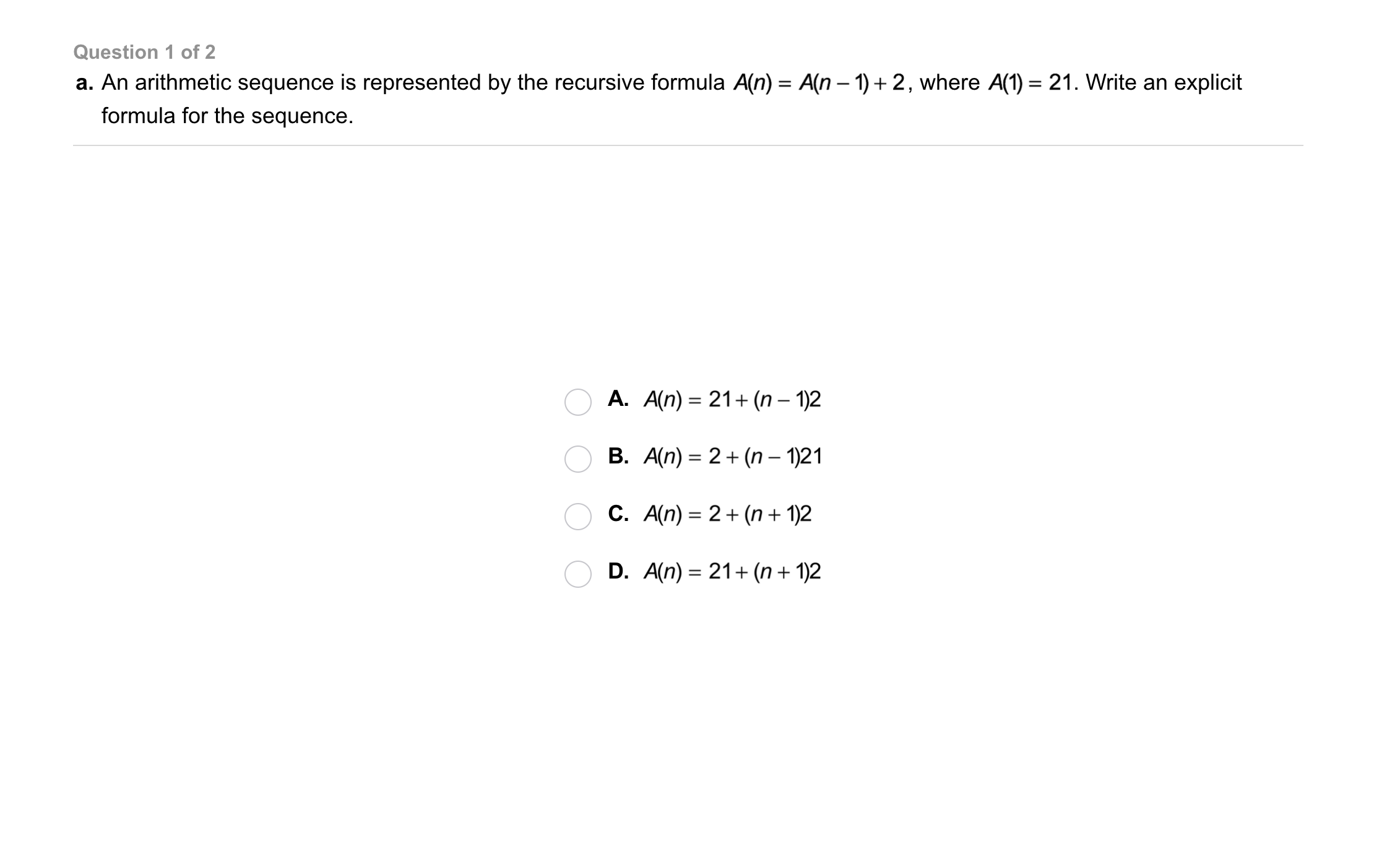21
21
10 pts
Problem 5 Got It?
A
B
C
D22
22
10 pts
Problem 5 Got It?
A
B
C
D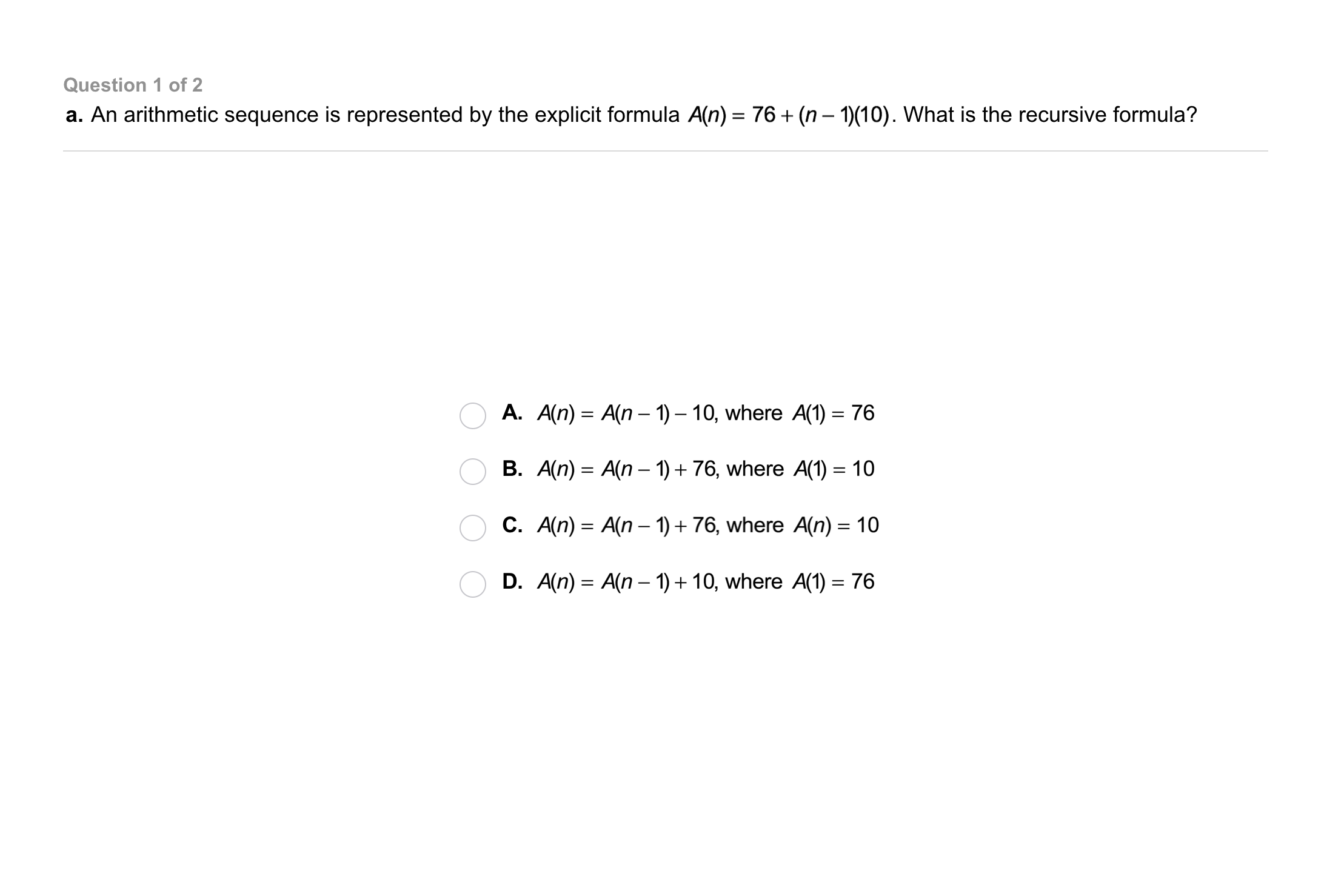23
23
10 pts
Problem 6 Got It?
A
B
C
D24
24
10 pts
Problem 6 Got It?
A
B
C
D25
25
10 pts
A
B
C
D26
26
10 pts
A
B
C
D27
27
10 pts
A
B
C
D28
28
10 pts
A
B
C
D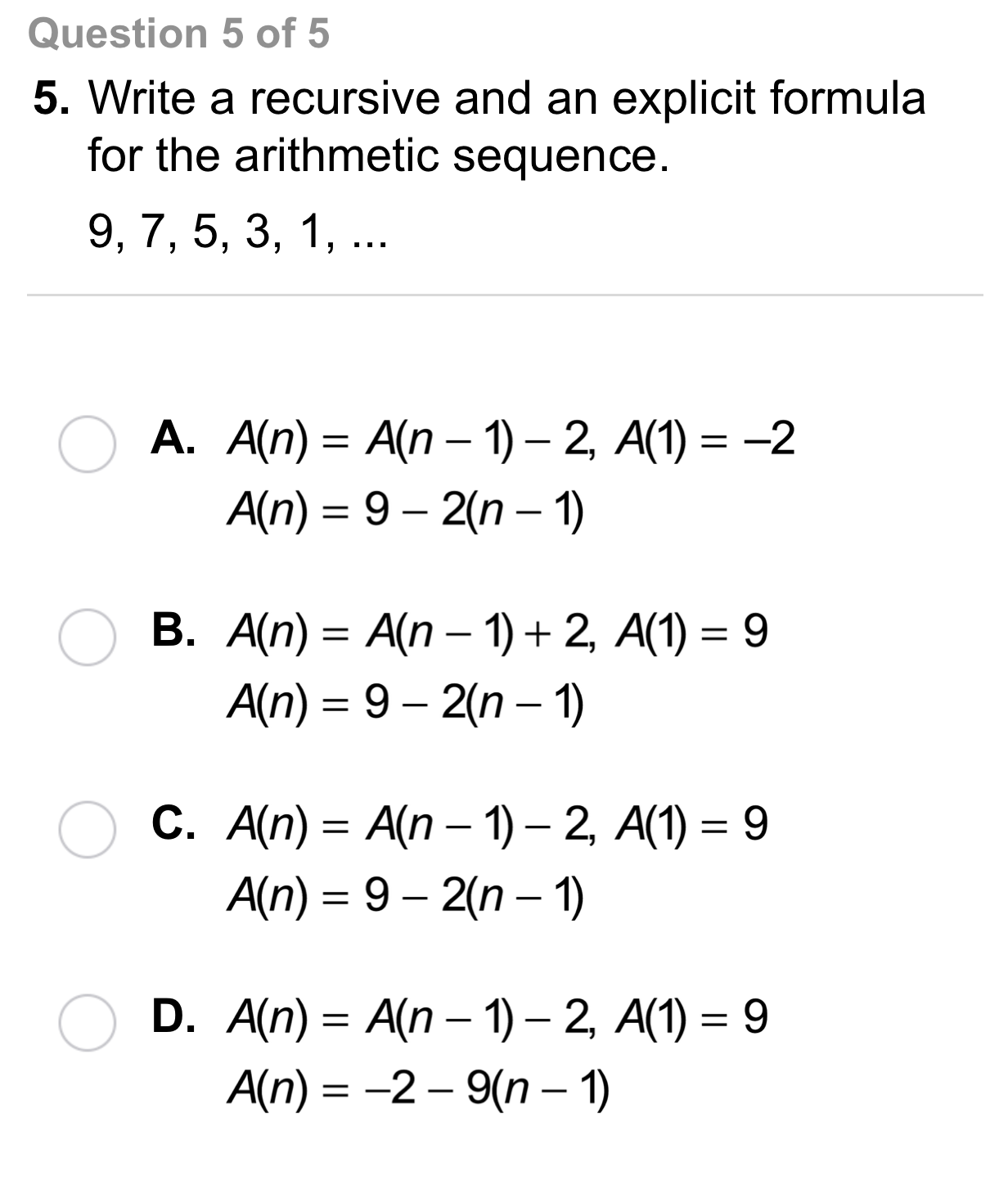29
29
10 pts
A
B
C
D30
10 pts
Vocabulary: Consider the following arithmetic sequence: 25, 19, 13, 7, ...
Is the common difference 6 or -6?
6
-6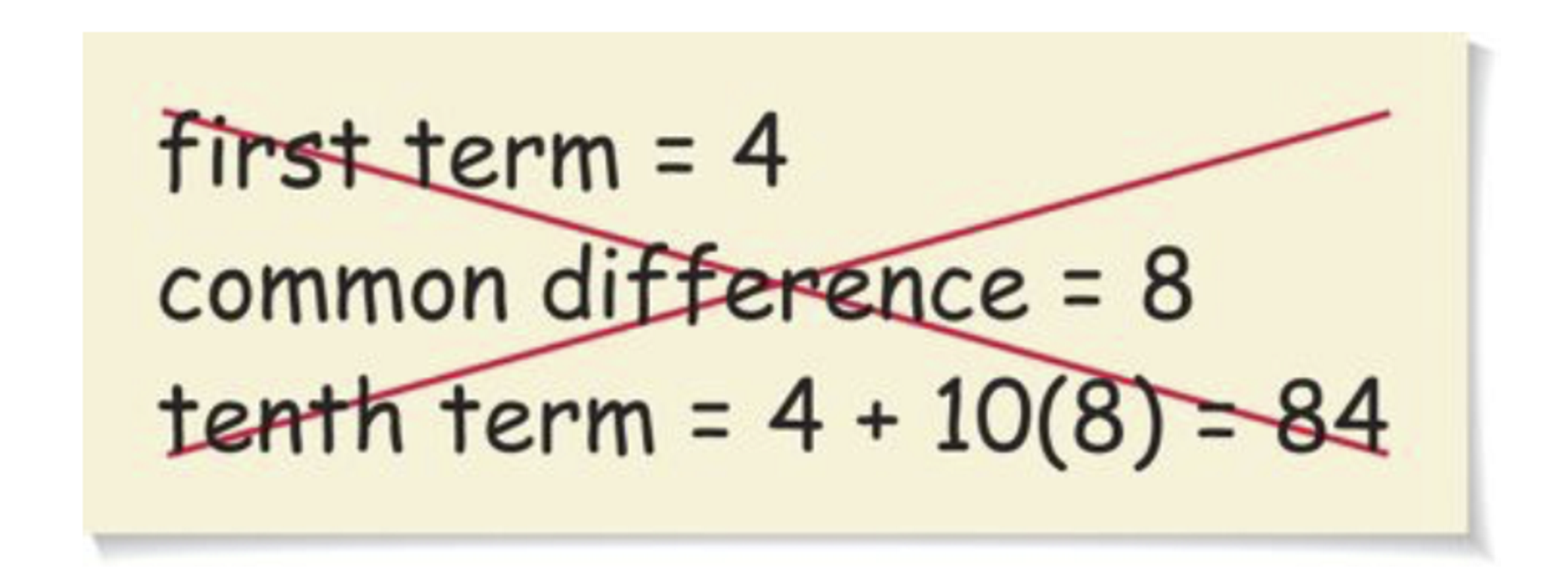32
32
10 pts
Error Analysis: Describe and correct the error in finding the tenth term of the arithmetic sequence 4, 12, 20, 28, ...33
10 pts
Review Lesson 4-6: Consider the function.
Match each domain element in the left column with its related range element in the right column. Use each domain element only once.
• -3
• -1.25
• 0
• 1
• 10
• 5
• -40
• 12
• 0
• -4
34
10 pts
Review Lesson 4-6: Consider the function.
Match each domain element in the left column with its related range element in the right column. Use each domain element only once.
• -3
• -1
• 0
• 1
• 10
• 300
• 0
• 27
• 3
35
10 pts
Review Lesson 2-6: Fill in the blanks.
• 5.25
• 480
• 132
• A pool fills at a rate of 8 gal/min. This is __?__ gal/h.
• A ball is thrown at a speed of 90 mi/h. This is __?__ ft/sec.
• You buy bottled water in 12-packs that cost \$3 each. If you drink 3 bottles per day, your cost is \$__?__ per week.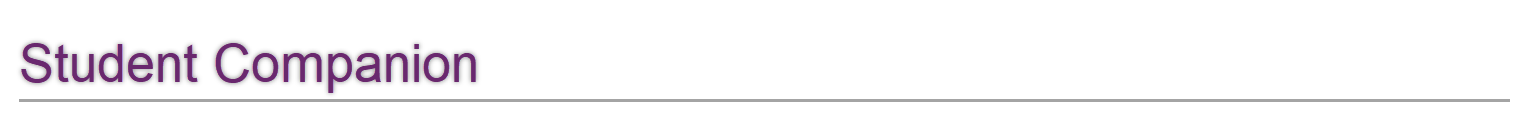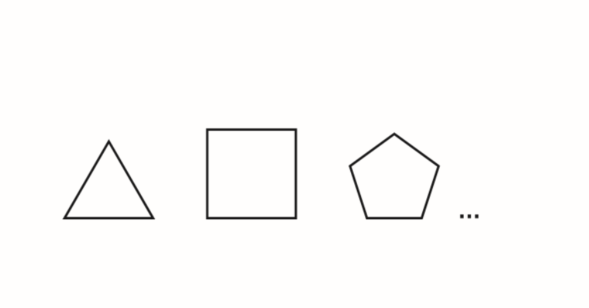36
36
5 pts
Vocabulary Review: What is the name of the next shape in the pattern shown?
circle
hexagon
rectangle
octagon
37
10 pts
Vocabulary Review: What is the next number in the pattern?

38
10 pts
Vocabulary Review: What is the next number in the pattern?

39
10 pts
Vocabulary Review: What is the next number in the pattern?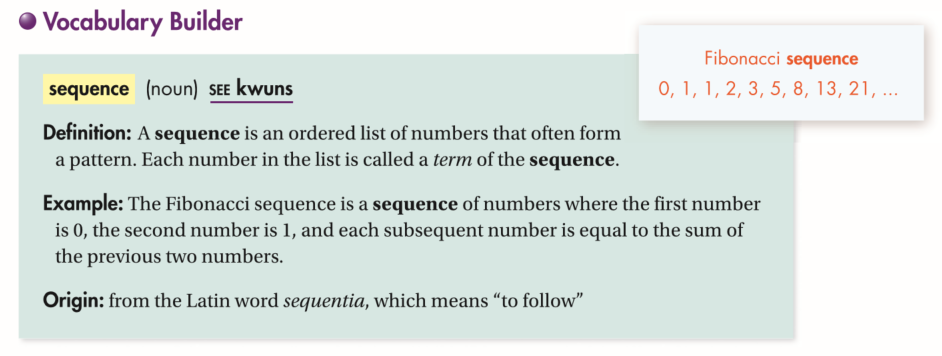40
10 pts
Use Your Vocabulary: The set is a sequence.

Explain the pattern.
41
100 pts
Notes: Take a clear picture or screenshot of your Cornell notes for this lesson. Upload it to the canvas. Zoom and pan as needed.

For a refresher on the Cornell note-taking system, click here.
42
10 pts
Reflection: Math Success
Add to my formatives list

Formative uses cookies to allow us to better understand how the site is used. By continuing to use this site, you consent to the Terms of Service and Privacy Policy.